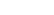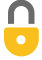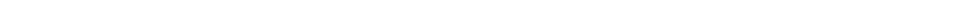Problem 7
Q

# A water heater can generate 32,000 kJ/h. How much water can it heat from $12 ^\circ \textrm{C}$ to $42 ^\circ \textrm{C}$ per hour?A
$250 \textrm{ kg/hr}$In order to watch this solution you need to have a subscription.VIDEO TRANSCRIPT

This is Giancoli Answers with Mr. Dychko. We're gonna take our usual formula for heat transfer, Q equals mc times change in temperature, and divide both sides by time. And we can solve for kilograms per hour or mass per time by dividing both sides of this by C Δ temperature. And that gives us mass per time is the heat per time divided by specific heat capacity times change in temperature. So, that's 32,000 kilojoules per hour or times 10 to the 3 joules per hour. And then divided by 4,186 joules per kilogram Celsius degree and then times by the change of temperature, final temperature of 42 degrees Celsius minus initial temperature of 12 degrees Celsius. And that gives about 250. And the units are kilograms per hour. The joules cancelled here the Celsius degrees cancelled here. And having 1 over kilograms in the nominator is the same as having kilograms in the numerator. And you can multiply top and bottom by kilograms to see how that works here. So, this is kilograms per hour or mass per time of water heated up.